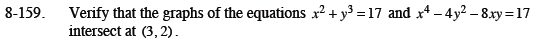Home > CCA2 > Chapter 8 > Lesson 8.3.2 > Problem8-159

8-159.

Verify that the graphs of the equations x2 + y3 = 17 and x4 − 4y2 − 8xy = 17 intersect at (3, 2). Homework Help ✎Insert the values of the point into the equations.

32 + 23 = 17

34 − 4(22) − 8(3)(2) = 17

Simplify.

9 + 8 = 17

81 − 16 − 48 = 17

17 = 17